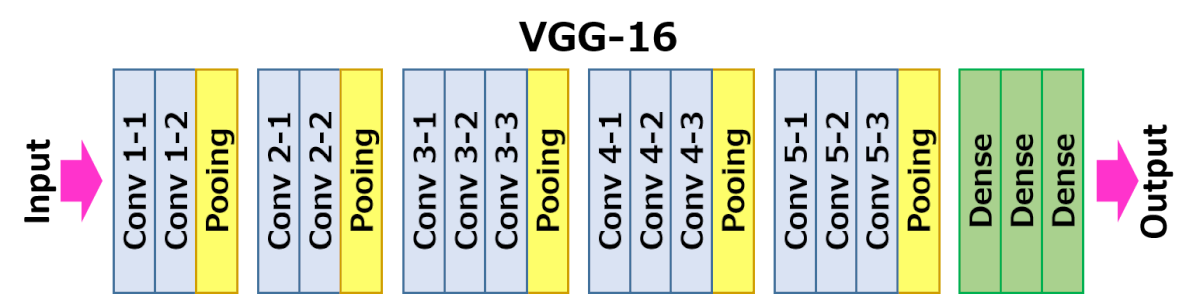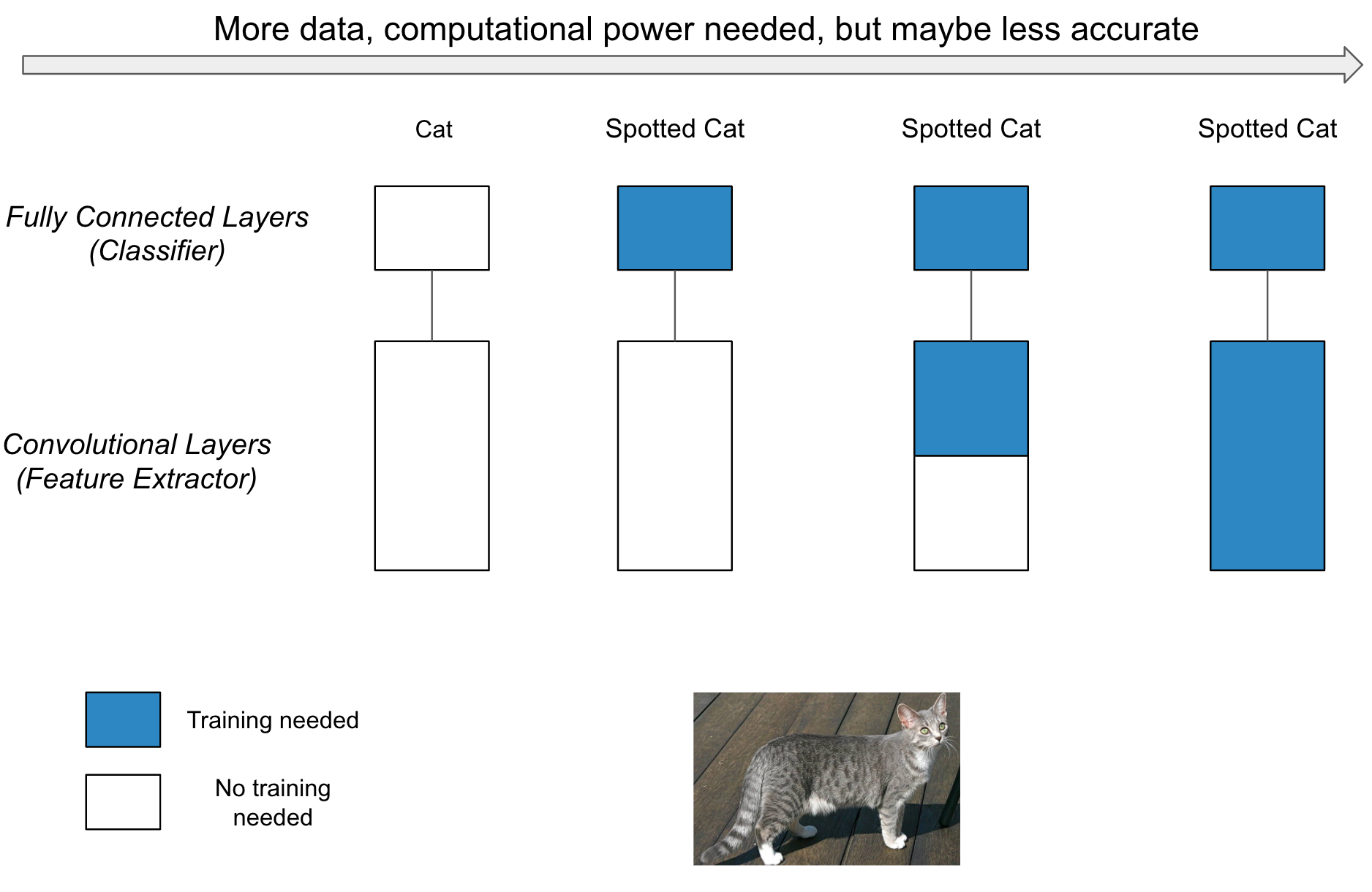# Transfer Learning

Posted by Xiaozhe Yao on June 12, 2020

Generally, transfer learning is a process where a model trained on one problem is used in some way on a second related problem. In deep learning, it refers to the technique whereby a deep learning model is first trained on a problem $P_1$, then one or more layers from the trained model are then used in a new model trained on the problem $P_2$ of our interest.

In Deep Learning(Page. 536), they mentioned that “this is typically understood in a supervised learning context, where the input is the same but the target maybe of different nature.”

For example, assume we have two tasks stated below:

• $P_1$: Classify images from the ImageNet dataset. ImageNet is a large dataset with over 14 million images in 1000 categories.
• $P_2$: Classify images from a hand-crafted dataset with only hundreds images in 10 categories.

In the above example, the inputs are the same images, but the target are different: In $P_1$, the prediction is a category in the 1000 categories, but in $P_2$, the prediction is a category in the 10 categories. Hence, we found that even though the inputs are the same, the predictions we want are different if we use a different neural network. Then the problem occurs: Do we need two different neural networks to handle those two tasks? The problem can be then divided into two parts:

• Do we need different neural network architectures?
• Do we need the weights in every layer to be different?

To answer these questions, we need to take a look at how the neural network works. We will take a convolutional neural network (more precisely, the VGG net) as an example. The architecture of VGG-16 are illustrated in Fig. 1, and we found it consists of only convolutional blocks (including the convolutional layers and pooling layers) and fully connected layers. In the previous article, we mentioned that the convolutional blocks can work as the feature extractor, while the fully connected layers are the actual classifiers. Hence, we conclude that there are two functional blocks in VGG-16 net: the feature extractor and classifier. The feature extractor is used to acquire the high-level features (for example, boundaries of objects) from the input image and the classifier is used to predict the category based on the high-level features.Fig.1 Illustration of VGG-16 Architecture. There are 5 convolutional blocks and 1 fully connected layers in the architecture.

One important assumption is that, with the same high-level features, the different classifiers can output different predictions. For example, in our above example, given a single cat image, our two tasks might be:

• $P_1$: Since the Imagenet dataset only includes a general cat category, we expect our deep learning model predict the image to be a cat.
• $P_2$: In $P_2$, we have 10 different types of cats, and we expect our deep learning model predict the image to be the exact type. For example, assume the ten types are of different colors.

Ideally, the feature extractor can extract the high-level feature that sufficiently represent the image. In our case, the output of feature extractor might be $(hairy, fat, spotted, with : tail)$. In different tasks, we will use the same feature and find out if the different classifiers can get different predictions.

With the first classifier in $P_1$, we can infer that the image represents a cat. The classifier make such a prediction because in the dataset, most objects with the property $(fat, hairy, with : tail)$ are cats. In $P_2$, we may have a different classifier which also takes the color into consideration. In this case, the prediction given by the classifier will be a spotted cat as the color feature is also captured.

From this simple example, we can answer the two questions above: We do not necessarily need a completely different neural network architecture as we can use the same architecture but with different weights to achieve the goal. Besides, we do not need the weights in every layer to be different. As long as the feature extractor can capture enough high-level features, we can change the classifier partially and then it can predict the image to our desired category.

Then there comes a new question: what if our feature extractor works well in the first case, but not in the second case? This can sometimes happen because if we consider the convolutional blocks as feature extractors, then the lower level convolutional layers (the layers that are closer to the input) will extract some low level, general features (such as lines, colors, etc) while higher level convolutional layers will extract some high level, specific features (such as hairy, boundaries, has tail or not, etc). More precisely, the features that we learned through a convolutional neural network are hierarchical. If the features are too specific, we may not be able to get a good classifier for our task. For example, with the same feature $(hairy, fat, spotted, with : tail)$, but our task is to identify what color of the cat is in the image. In this case, no matter how we tried to train our classifier, it will not work very well. Therefore, in some cases, we still need to train the convolutional layers. However, we can still reuse some low-level convolutional layers.

To conclude, we can have four strategies when facing a new deep learning task as illustrated in Fig. 2, from left to right they are:

• Use the pretrained model directly. This strategy usually not happens, because in most cases, our task is somehow different from the task where the pretrained model was trained. In this case, the output of the pretrained model may not be what we need (for example, here we expect our model predict the image to be a ‘spotted cat’, not a ‘cat’).
• Train the classifier only and leave the convolutional layers fixed. This is an extreme case where we use the deep learning model as a feature extractor and build our own classifier. We will usually use this strategy when our computational power is limited, the dataset that we have is small, or the pretrained model solves a problem that are similar to the ones they we are interested in.
• Train some high-level convolutional layers and the classifier, leave others fixed.
• Train the entire model. In this case, we will use the architecture in the deep learning model but not the weights at all and train it according to our dataset. The model is learning from scratch, and will take a longer time and need a larger dataset to finish. Even though we trained it from scratch, sometimes the accuracy is still lower than using pretrained models if the dataset in $P_2$ cannot provide good features (Here good features refer to the features that have similar patterns with the images when we actually serve the model.).Fig.2 Illustration of different strategies for transfer learning.

## Summary

In this section, we introduce the transfer learning in more detail. By using transfer learning, we could achieve higher accuracy with less computational power and dataset. The computational power and dataset are two bottlenecks for deep learning applications, and since transfer learning can tackle the problem, it soon becomes the de facto best practices in deep learning tasks. The key idea behind transfer learning is simple: fix some generic, problem-agnostic layers, and train other problem-specific layers on new dataset.

Even though the idea and implementation of transfer learning is simple and straightforward, it needs to be pointed out that the use of transfer learning is wide and promising. It is widely used as most data scientists are now using pretrained models open-sourced by big companies and leading research institutes rather than build it from scratch because it saves costs and time. At the same time, big companies and research institutes are willing to open source their pretrained models trained on extremely huge dataset. For example, Tencent released its largest multi-label image dataset named Tencent ML Images in 2019 with 20 million images and 10 thousands categories. It is promising because the idea behind transfer learning: extract the generic knowledge from the data, and then apply it somewhere else, seems to be (though there’s no evidence to prove this yet) important to achieve general intelligence.